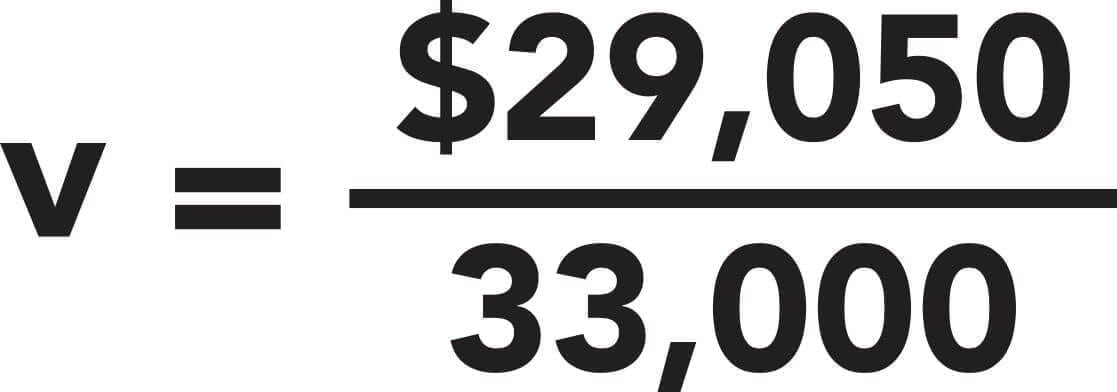# What Are Fixed Costs?Fixed costs are business costs that do not change with an increase or decrease in output.

They are the same no matter how many goods are produced or how many services are delivered. Costs that do change with output are known as variable costs.

Together, these costs paint an accurate picture of business expenses.

## What Is Included in Fixed Costs?

Because fixed costs are not directly associated with production, they are referred to in accounting as indirect costs.

Fixed costs make up the overhead costs of a business and are often time-related. The following are examples of fixed costs:

• Rent
• Equipment leases
• Insurance
• Property taxes
• Interest
• Loan payments
• Salaries (not hourly labor)
• Depreciation
• Phone, internet, and other communication costs

In each of these examples, the costs are incurred regardless of the level of output the business has; therefore, they are classified as fixed costs.

## Why Track Fixed Costs?

Tracking costs in a business is absolutely crucial to managing the financial health of the business and setting appropriate, achievable goals.

Understanding your fixed and variable costs is also an important first step in calculating the break-even point for your business.

### The Break-Even Point

The break-even point is the point at which revenues exceed total variable and fixed costs.

Until the break-even point is reached, no revenue generates profit—it is still less than total costs.

Calculating the break-even point is fairly straightforward, but it does require that all costs are first tracked and appropriately classified.

## Calculating the Break-Even Point in Units

Calculating your break-even point consists of three steps. First, it is important that your costs have been tracked and appropriately classified.

Let’s use data from the sample table below.

From the table above, we can see that there are both variable and fixed costs. Only the last two entries are fixed costs—the rest are variable.

### Using the Break-Even Formula

The break-even formula divides total fixed costs by the revenue per unit, with the variable cost per unit subtracted.

Totaling the fixed costs from our sample table is easy. The “variable cost per unit” part of the formula is also fairly straightforward; the formula is as follows:

Not sure how to handle variable costs? Check out this summary.

Let’s start filling in some blanks.

If the quantity of units produced in the period is 33,000 (this is the value of Q) and the revenue per unit is \$3.99, we have all the information we need to determine the break-even point in units for our sample data set.

### Calculating Variable Cost Per Unit

The first step is to find our total variable costs for the period. You can see in the image below that the sum of variable costs is \$29,050.

Then we plug what we know into the unit variable cost equation.This results in a variable cost per unit of \$0.88. Now we have part of the information we need to calculate our break-even point.

### Using Fixed Costs in the Break-Even Formula

We’re almost there. Now all we need is the total fixed costs and we’ll be ready to calculate the break-even point in units.

Having calculated total fixed costs of \$2,950 for the period, we’re ready to plug everything into the break-even formula.The result is 948.55.

Since we likely can’t sell half a unit, we’ll round that up to 949 units.

This means that, to turn a profit, our sample company needs to sell at least 949 units in a period.

Since the sample data was from a single month’s reporting, then our 949-unit calculation means that our sample company needs to sell that many units in a month to turn a profit.

Calculating your business’s break-even point in units isn’t the only way to determine what point your business needs to reach to start turning a profit within a specific period.

Use this link to explore more ways to model your business needs and discover exactly where you will start making a profit.

• Email
• More Networks
[class^="wpforms-"]
[class^="wpforms-"]
[class^="wpforms-"]
[class^="wpforms-"]
[class^="wpforms-"]
[class^="wpforms-"]
[class^="wpforms-"]
[class^="wpforms-"]
[class^="wpforms-"]
[class^="wpforms-"]
[class^="wpforms-"]
[class^="wpforms-"]
[class^="wpforms-"]
[class^="wpforms-"]
[class^="wpforms-"]
[class^="wpforms-"]
[class^="wpforms-"]
[class^="wpforms-"]
[class^="wpforms-"]
[class^="wpforms-"]
[523.251,1046.50]
[523.251,1046.50]
[523.251,1046.50]
[523.251,1046.50]
[523.251,1046.50]
[523.251,1046.50]
Share via